# Dependent Samples - Quantum Column Means Test

By default, when this test is selected Q computes standard errors using Taylor series linearization. To replicate the results of IBM SPSS Data Collection Model programs (e.g., Survey Reporter), you need to also change the Weights and significance to Kish approximation in Statistical Assumptions.

Where Taylor series linearization is performed, and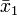$\bar x_1$ and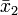$\bar x_2$ are the two means, the test statistic is: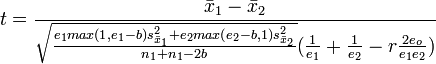$t=\frac{\bar x_1-\bar x_2}{\sqrt{\frac{e_1 max(1, e_1-b)s^2_{\bar x_1} + e_2 max(e_2-b, 1)s^2_{\bar x_2}}{n_{1} + n_{1} - 2b}}(\frac{1}{e_1}+\frac{1}{e_2}-r \frac{2e_o}{e_1 e_2})}$

Otherwise, the test statistic is: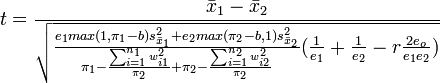$t=\frac{\bar x_1-\bar x_2}{\sqrt{\frac{e_1 max(1, \pi_1-b)s^2_{\bar x_1} + e_2 max(\pi_2-b, 1)s^2_{\bar x_2}}{\pi_1 - \frac{\sum^{n_{1}}_{i=1} w^2_{i1}}{\pi_2} + \pi_2 - \frac{\sum^{n_{2}}_{i=1} w^2_{i2}}{\pi_2}}(\frac{1}{e_1}+\frac{1}{e_2}-r \frac{2e_o}{e_1 e_2})}}$

where:$1$ and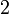$2$ refer to the two variables and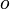$o$ to the overlap,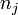$n_j$ is the sample size,$e_j$ is the effective sample size,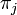$\pi_j$ is the weighted sample size,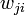$w_{ji}$ is the weight for the$i$th observation in the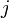$j$th group,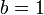$b=1$ if Bessel's correction is selected and 0 otherwise,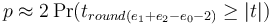$p \approx 2 \Pr(t_{round( e_1 + e_2 - e_0 - 2)} \ge |t|)$,$r$ is Pearson's Product Moment Correlation for the overlapping sample,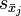$s_{\bar x_j}$ is the Standard Error of the mean,
the p is returned as NaN if the denominator is less than 0.00001.

Also note that if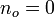$n_o = 0$ the Independent version of the test is conducted instead.